A Quadratic Equation looks like this:And it can be solved using the Quadratic Formula:That formula looks like magic, but you can follow the steps to see how it comes about.

## 1. Complete the Square

ax2 + bx + c has "x" in it twice, which is hard to solve.

But there is a way to rearrange it so that "x" only appears once. It is called Completing the Square (please read that first!).

Our aim is to get something like x2 + 2dx + d2, which can then be simplified to (x+d)2

So, let's go:

 Start with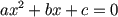Divide the equation by aPut c/a on other side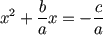Add (b/2a)2 to both sides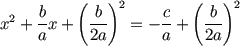The left hand side is now in the x2 + 2dx + d2 format, where "d" is "b/2a" So we can re-write it this way: "Complete the Square"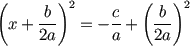Now x only appears once and we are making progress.

## 2. Now Solve For "x"

Now we just need to rearrange the equation to leave "x" on the left

 Start withSquare rootMove b/2a to right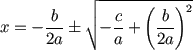That is actually solved! But let's simplify it a bit: Multiply right by 2a/2a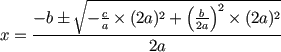Simplify:Which is the Quadratic formula we all know and love: